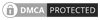## Online K-factor calculator for fire sprinklers

To find the flow from a sprinkler head or a water mist nozzle, we need to use the K-factor formula. This is the starting point for most water-based fire protection design and calculations. We can use this formula for any device for which we have a K-factor, and that can be a sprinkler, nozzle, water cannon, foam pourer and may other devices.

You will find below an on-line K-factor calculator which you can use to calculate any of the three variables in the K-factor equation. It can be used for both fire sprinkler and water mist system or other types of heads & nozzles. For a detailed description of please see K-Factor formula for fire sprinklers.

### How to use the K-factor calculator

You will see that we have a metric and imperial version available. This is not strictly necessary as the formula is the same so as long as you use the correct units, it's not an issue, however having the units added clarity.

If you look at the calculator, you will see Flow, Pressure and K-factor across the top. These are the three K-factor variables which we can calculate. Under each are the two parameters which we need to specify and directly below. Let's take an example we wish to find the flow from a fire sprinkler head, and we know the K-factor is 60.8 and the pressure to the head is 0.68 Bar then the flow from the sprinkler will be 50.14 L/min, this is illustrated below:Canute LLP
15 Queen Square
Leeds, West Yorkshire
United Kingdom LS2 8AJ

t: +44 (0) 113 328 0350
e: info@canutesoft.com

 Copyright © 2021, Canute LLPRegistered in England & Wales, Partnership No. OC305985